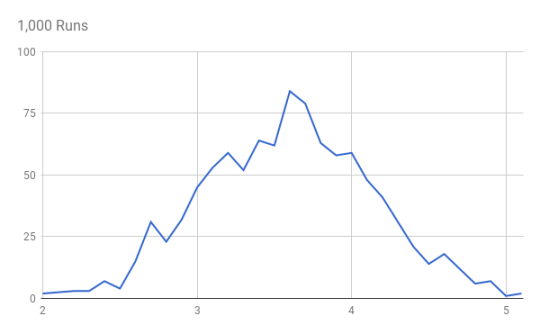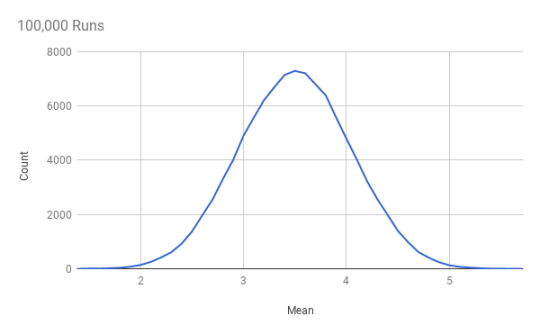# Uncertainty Wednesday: Sample Mean

Last Uncertainty Wednesday I introduced a definition of climate as the probability distribution over weather states. That post ended with a question about how historically observed statistics relate to this distribution. I am in Vienna Austria for a conference and the temperature yesterday got up to 70 degrees (Fahrenheit). Intellicast shows historic average high temperature for September 27 to be 65 degrees (unfortunately not saying how many years of data are averaged which is something I will try to track down). So clearly yesterday’s high is above the average high observed for this day in the past. But what should we conclude from that?

To get there we will first learn a bit more about the relationship between the expected value of a distribution and the observed sample means. As I had pointed out in my original post on expected value, this is the source of a great deal of confusion.than the complexity of weather, let’s take a really simple probability distribution: rolling a fair six-sided die. The probability distribution is really simple. Each of the values 1, 2, 3, 4, 5 and 6 has equal probability of 1/6. Hence

EV = 1/6 * (1 + 2 + 3 + 4 + 5 + 6) = 1/6 * 21 = 3.5

Now let’s look at a variety of samples from this distribution and the observed sample mean. To look at this I wrote some hacky Python code which you can see here (which I stored in a file called samplemean.py):

from __future__ import division
from random import randint
import sys

runs = int(sys.argv)
dist = {}

size = int(sys.argv)
total = 0

for run in range(0, runs):

total = 0
for i in range (0, size):
r = randint(1, 6)
# print i+1, “: ”, r
total += r
# print “Sample mean: ”, total / size

mean = total / size
if mean in dist:
dist[mean] += 1
else:
dist[mean] = 1

for mean in sorted(dist):
print “%s: %s” % (mean, dist[mean]);

The inner loop creates a sample of size provided in the second command line argument and computes the mean. The outer loop runs that as many times as provided in the firs command line argument. So python samplemean.py 1000 10 for instance takes 1,000 samples of size 10 each and computes their respective means and counts how many time each mean occurs.

Here is the distribution of sample means for sample size 10 that results from that for different numbers of runs (I took the output from the above program, pasted it into Google Sheets to graph it):

What is going on here? What we are seeing is that the sample mean for a small sample of size 10 can vary a lot. In fact in 100,000 runs we see sample means very close to 1 (meaning most rolls came up as a 1) and some very close to 6 (meaning most rolls came up as a 6). As we graph the sample means for few runs they come out with a lot of their own variance. But as we draw a lot of them they seem to have the shape of a normal distribution.

Next Wednesday we will dig deeper into this phenomenon. And we will also look at what happens when we increase the size of our samples.